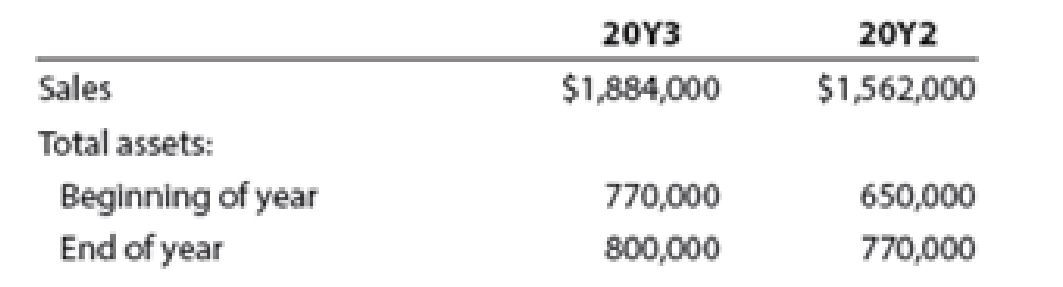# Asset turnover ratio Financial statement data for years ended December 31, 20Y3 and 20Y2, for Edison Company follow: a. Determine the asset turnover ratio for 20Y3 and 20Y2. b. Is the change in the asset turnover ratio from 20Y2 to 20Y3 favorable or unfavorable?### Financial And Managerial Accounting

15th Edition
WARREN + 1 other
Publisher: Cengage Learning,
ISBN: 9781337902663

#### Solutions

Chapter
Section### Financial And Managerial Accounting

15th Edition
WARREN + 1 other
Publisher: Cengage Learning,
ISBN: 9781337902663
Chapter 5, Problem 7BE
Textbook Problem
829 views

## Asset turnover ratioFinancial statement data for years ended December 31, 20Y3 and 20Y2, for Edison Company follow:a.    Determine the asset turnover ratio for 20Y3 and 20Y2.b.    Is the change in the asset turnover ratio from 20Y2 to 20Y3 favorable or unfavorable?

a.

To determine

Determine the asset turnover for 20Y3 and 20Y2 for Company E.

### Explanation of Solution

Asset turnover ratio is used to determine the efficiency of the company towards use of asset towards generation of sales. The formula to calculate asset turnover is given below:

Assetturnover=NetrevenueAverage total assets

Given information for 20Y3:

Sales/ net revenue = $1,884,000 Beginning assets =$770,000

Ending assets = $800,000 First, calculate the average total assets for 20Y3. Averagetotalassets=Beginningassets+Endingassets2=$770,000+$800,0002=$785,000

Now, calculate the asset turnover for 20Y3.

Assetturnover=NetrevenueAverage total assets=$1,884,000$785,000=2

b.

To determine

Comment whether the change in the asset turnover from 20Y2 to 20Y3 indicate a favorable or an unfavorable trend.

### Still sussing out bartleby?

Check out a sample textbook solution.

See a sample solution

#### The Solution to Your Study Problems

Bartleby provides explanations to thousands of textbook problems written by our experts, many with advanced degrees!

Get Started

Find more solutions based on key concepts
Describe this textbooks three themes.

Accounting Information Systems